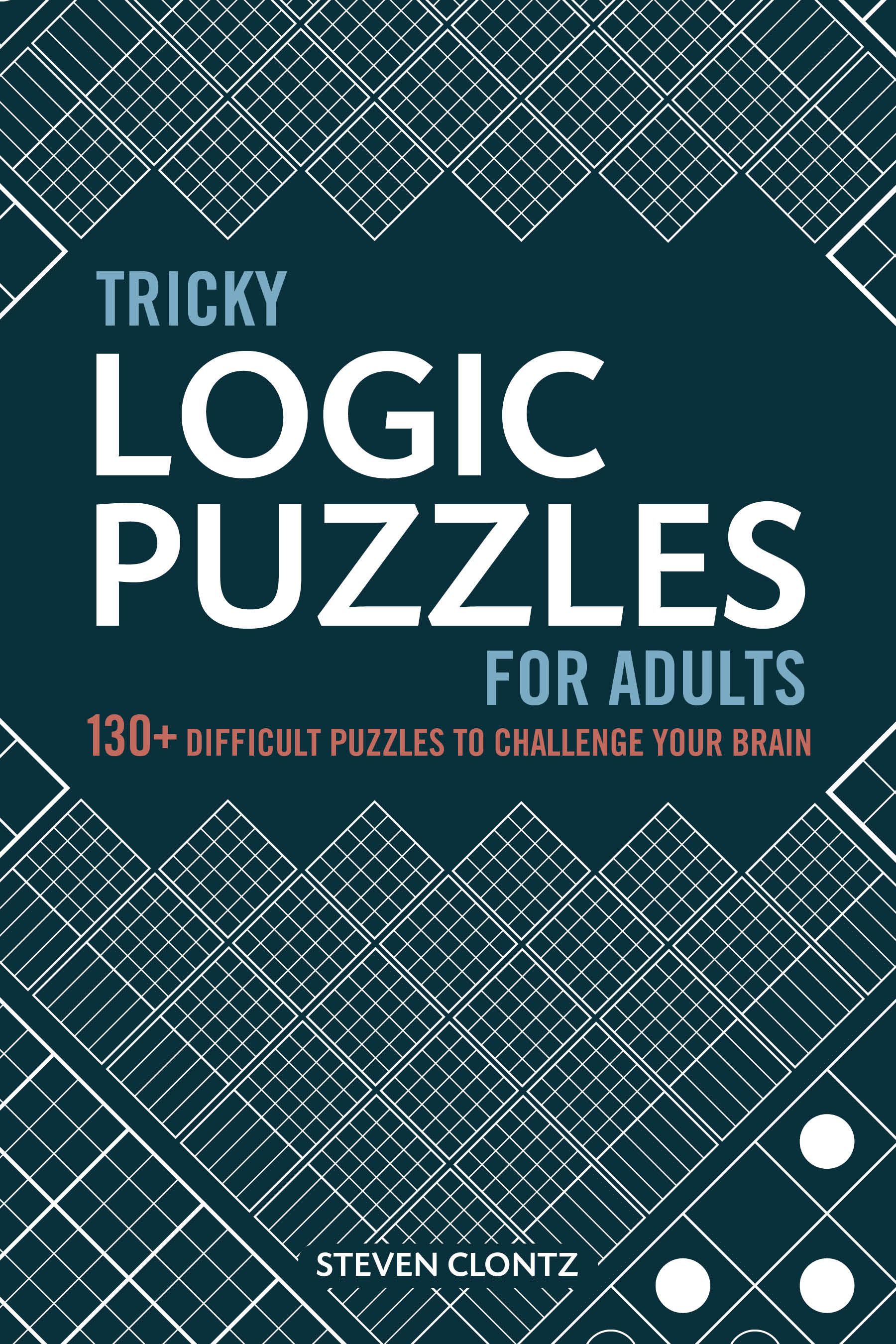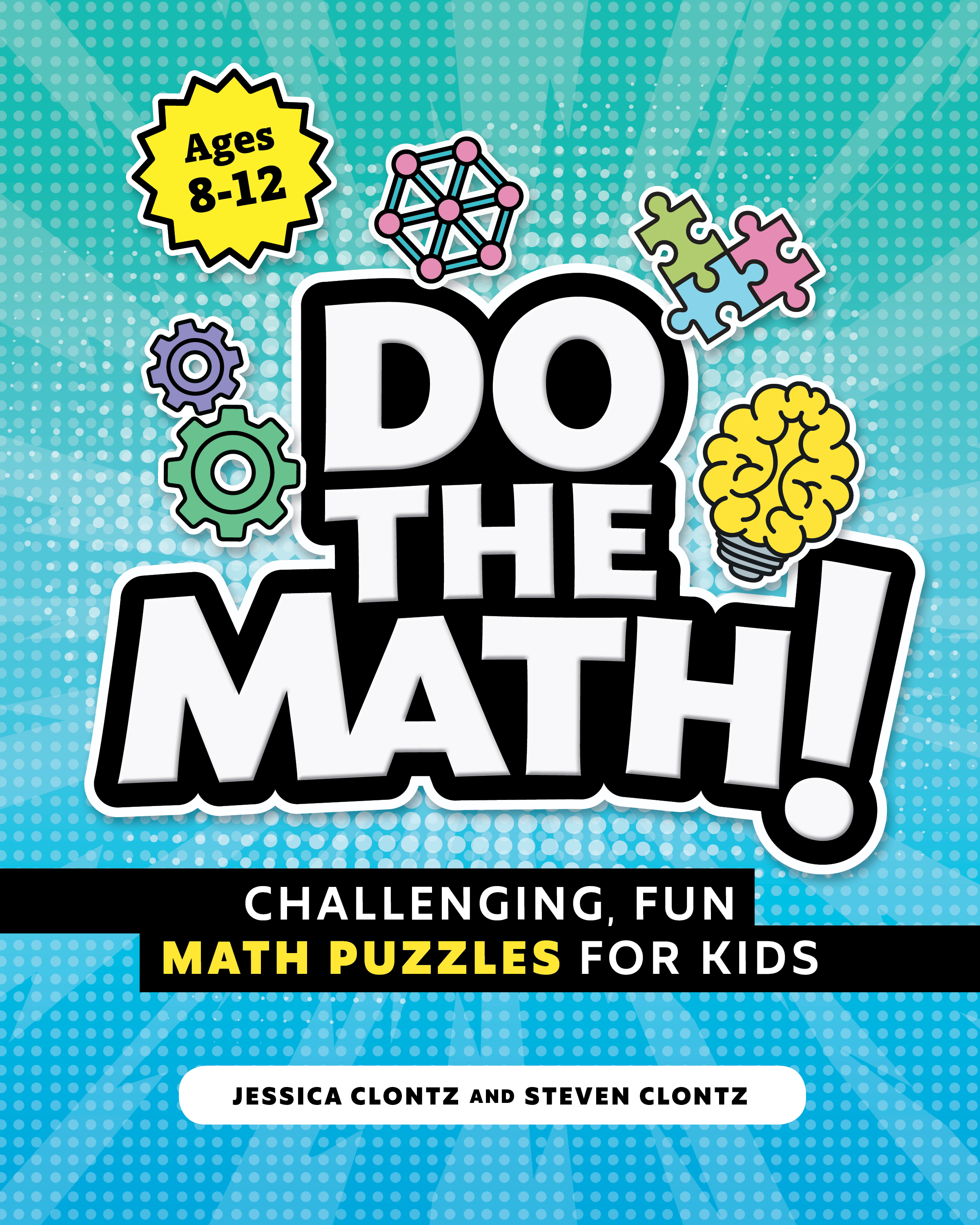# Puzzle Types

math, word, logic, and more

Generally speaking, a puzzle is any task which satisfies two properties:

• It is designed to entertain the solver.
• There is a well-defined solution.

This can be accomplished through many methods! Here are a few such categories of puzzle types that I’ve come across in my work.

Psst. I heard you like puzzles? Try out my new free logic puzzle game at SumIt.clontz.org. It works on your phone or web browser without having to install anything.

## Cryptic puzzles

Example: the first two puzzles on my puzzle homepage.

These are the most common type of puzzles found in quality puzzlehunts and escape rooms, and enthusiasts consider these challenges to be simply “puzzles” Such puzzles might alternatively be called “designer” puzzles which are meant to be solved at most once by a given person, since part of the puzzle is the epiphany required to see an extraction technique. Note that “cryptic crosswords” (often just called “cryptics”) are a type of word puzzle that doesn’t really fit this definition (although the overlap of cryptic puzzle and cryptic crossword fans is non-trivial!).

In a cryptic puzzle, the ultimate goal/answer typically should be well-defined (i.e. enter a certain word or phrase into an online form which is accepted; find a clue to a location where a previously designated token can be found). However, the mechanics of extracting this solution are left partially or completely vague, and it’s up to the solver to guess at several interpretations of what the puzzle could mean until one fits. Often the puzzle’s title or its flavortext can hide clues to this extraction: “you’d be blind to not know how to solve this” might be a (not-so) subtle clue to look for a way to find Braille letters in the puzzle.

Designing such puzzles takes a lot of practice, in addition to knowledge of your audience. Different communities expect different levels of difficulty, and different players will have different levels of “genre savviness”. These are by far my favorite type of puzzle to design and solve, but it’s hard to tell at a quick glance if such a puzzle is a high-quality difficult cryptic puzzle, or just badly written.

## Logic Puzzles

Examples include Sudoku, nonogram, calcudoku, Masyu, and logic grid puzzles.

A logic puzzle is typically characterized by a grid which must be completed by following some well-defined rules. Generally such puzzles have a unique solution (a property which itself helps the solver filling the grid). Often these puzzles may be made easier by partially filling out the puzzle for the solver. I’m a particular fan of picross puzzles (also called nonograms) because the solution paints a picture (i.e. gives the solver new information unrelated to the properties of the puzzle itself).

## Math Puzzles

Arguably, these come in two varieties.

### Explicit

Given $$xy=6$$, $$xz=10$$, and $$yz=15$$, find the value of $$xyz$$.

What distingishes these from math problems in that a math puzzle is meant to be recreational, or perhaps competitive. These puzzles typically don’t lend themselves to algorithmic solutions; at least, not algorithms which are traditionally taught in school, anyway. See also: AMC comeptitions, Putnam problems, and other traditional math competitions.

By the way, the trick in the example is to not try to solve for xyz, but first try to solve for x^2y^2z^2… It’s a fun puzzle the first time you hear it, but the top competitors in these competitions memorize hundreds of tricks just like that. So ironically, while these puzzles are typically designed to challenge students’ mathematical abilities, the optimal strategy for solving such puzzles is to memorize a large backlog of tricks and gimmicks, and figure out what slight variation is required for the puzzle at-hand.

### Implicit

You’ve developed a new social networking platform, and you plan to support 100 users for your beta release. Each user can be “friends” with every other user. How many “friendships” does your social network’s database need to be able to store?

Rather than explicitly asking a mathematical “solve for $$x$$” question, a modern math puzzle paints a narrative, and leaves the application of mathematics up to the solver.

For instance, since the first user could have up to 99 friends, the second user could have up to 98 other friends, and so on, we find that the answer is:

$99+98+\dots +2+1=4950$

But that might be hard (and we could make it completely infeasible by upping the number to a thousand, or million users). In that case, players are forced into making the clever observation that each of the 100 users can have 99 other friends, but since that’s double-counting friendships we should divide this product by two:

$\frac{100\times 99}{2}=4950$

Of course, if you know some graph theory, you could also approach this problem by expressing it as a graph and asking yourself “how many edges are in the complete graph with 100 vertices?”. This illustrates that a good modern math puzzle can both challenge and teach mathematics, without explicitly being posed as a “solve for $$x$$” math problem.

It’s worth noting that this puzzle is very close to being a mathematical “word problem”. And if it was given in the context of a course on combinatorics, it would be! But unless you were taught about triangular numbers in class just yesterday, the point of the challenge isn’t to test your memorization of the formula $$\sum_{k=0}^n k=\frac{n(n+1)}{2}$$, but to challenge your problem-solving and mathematical modeling abilities to come up with your own patterns and formulas.

## Mechanical Puzzles

Examples include jigsaw puzzles, nail puzzles, Rubik’s Cubes.

Probably the most common variant of puzzle; I expect most puzzlers consider a jigsaw piece as the “logo” for puzzling. These can be solved through trial-and-error, but particularly for Rubik’s cube-type puzzles there can be mathematical/logical methods for a solution as well.

## Trivia puzzles

Similar to cryptic puzzles, trivia puzzles usually have custom designs (otherwise, they’re just plain trivia). While trivia puzzles often have cryptic elements, such a puzzle may also be explicit (in which case, it might also be a type of word puzzle). In any case, the player must have some domain knowledge to solve such puzzles, or the ability to learn about the topic.

Novice escape room designers often fall into the trap of including trivia puzzles in their challenges, which would be very disappointing for a player stuck in a room for 60 minutes just because they were rusty on (say) ABBA’s discography! However, I often find myself enjoying trivia puzzles even when they involve domains I’m not very familiar with: just make sure I have a way to look up the solutions as needed, and preferably have a secondary challenge beyond the trivia once I’ve incorporated the solutions.

## Word puzzles

Examples include crosswords, Boggle.

Word puzzles are puzzles which require knowledge of a language. Most word puzzles are actually mathematical in nature assuming a dictionary is available; for example, there are 120 ways to permute the letters in a five-letter word without repeated letters, and there are 104 possible three-letter words in a 4 by 4 grid of letters.

The line gets blurred a bit with crossword puzzles; technically (as was pointed out by fellow puzzler Christopher Night) crosswords may have multiple answers and are up to interpretation (like pattern puzzles). In general, word puzzles which are based on defintions have a bit of flexibility (unless there’s a way to check the solution), so designing them takes finesse.

On the other hand, puzzles like Places Please are actually logic puzzles, even though they use words! This is because no prior knowledge of words is required, as they are all provided. Instead, players must simply use logic to find how these words can be used to fill out the grid.

A couple related concepts:

## Pattern Guessing

Example:

What comes next in the list? 1, 2, 4, …

These is not typically well-defined puzzles. Especially egregious are “which picture comes next in the pattern” Mensa-type questions. I’ll point out that in the example, both $$f(n)=2^n$$ and $$g(n)=\frac{n^2+n+2}{2}$$ satisify the requirements $$f(0)=g(0)=1,f(1)=g(1)=2,f(2)=g(2)=4$$, yielding equally reasonable guesses of $$f(3)=8$$ and $$g(3)=7$$, respectively.

## Riddles

Example:

A man and his son are involved in a car accident. The father dies, but the son is taken to the hospital. The doctor refuses to operate, saying “I cannot operate on my son!” How can this be?

Riddles don’t fit my definition of a puzzle since there’s no definite logically-deduced solution. Note that there are a few answers to the above riddle: “The doctor is the mother!” “The son has two fathers!” etc. etc. These may be fun to ponder in a low-stakes environment, but are bad form in any sort of competition such as puzzlehunts or escape rooms.